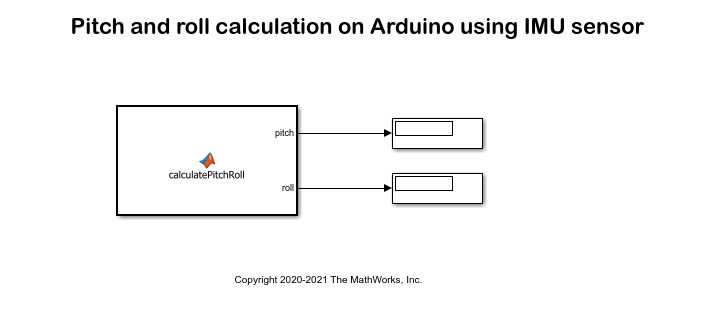Main Content

# Pitch and Roll Calculation on Arduino Using IMU Sensor

This example shows how to read the acceleration and angular velocity data from IMU sensor mounted on Arduino® hardware and calculate the pitch and roll angles.

### Prerequisites

Before you start this example, install these MathWorks® products:

• MATLAB

• Simulink

• Simulink Support Package for Arduino® Hardware

• MATLAB Support Package for Arduino® Hardware

### Hardware Required

• Arduino (any Arduino hardware with I2C peripheral that supports codegen from MATLAB Function block)

• MPU9250 (any IMU sensor with accelerometer and gryoscope)

Note: Any IMU sensor that supports codegen from MATLAB function block can be used in this example. If any other sensor is used to create IMU sensor object, for example if LSM9DS1 sensor is used, then the object creation needs to be modified to lsm9ds1(a) from mpu9250(a).

### Model

Simulink Support Package for Arduino hardware provides a pre-configured model that you can use to read the acceleration and angular velocity data from IMU sensor mounted on Arduino hardware and calculate the pitch and roll angles.

To open the model, run this command at the MATLAB command prompt:

```open_system('arduino_imu_pitch_roll_calculation'); ```### Algorithm

The alignment of the sensor axes should be as following:

• X-axis = Longitudinal axis or roll axis

• Y-axis = Transverse axis or pitch axis

• Z-axis = Vertical axis or yaw axis

In this model pitch and roll angle is calculated using accelerometer and gyroscope readings. After deploying the model on hardware, you can find out the pitch and roll angle based on the orientation of the sensor. The range of pitch and roll angle is [-90 degree, 90 degree]. If the sensor is kept steady and parallel to ground, i.e z-axis perpendicular to ground, both the readings should be 0. The pitch angle is positive for anticlockwise rotation with respect to Pitch axis and negative for clockwise rotation. For roll angle it is the same. To filter out noise at the beginning of the execution of the program, when the sensor is kept steady on ground with Z-axis being perpendicular to ground, the sensor is read multiple times and offset values for pitch and roll angle are calculated. These values are subtracted later when pitch and roll angle is calculated at every step. This removes steady noise from sensor readings to some extent.

### Configure and Run the Model on Arduino Board

1. Open the arduino_imu_pitch_roll_calculation model.

2. On the Hardware tab, click Hardware Settings to open the Configuration Parameters dialog box.

3. Select the Hardware Implementation pane and select your Arduino hardware from the Hardware board parameter list. Do not change any other settings. Click OK.

4. Click the Deploy to Hardware button to run this model on the Arduino board.

5. To view the output in MATLAB, run the model using the Monitor and Tune feature. To do this, go to the Hardware tab and click Monitor & Tune.

## Support

#### Model-Based Design for Embedded Control Systems

Download white paper